# Low Pass Filter how to? The sites on the internet seems to be wrong!

#### babaliaris

Joined Nov 19, 2019
158
Sites like this one, inform that in order to create a low-pass filter you can choose a small capacitance for the capacitor and then use the formula:
$R = \frac{1}{2πF_{c}C}$

in order to figure out the value of R.

Choosing a low C for the capacitor makes sense since $$Z_{C} = \frac{1}{jωC}$$ if C << 1 Z->infinity and acts as an open circuit, thus the output voltage is equal to the input. While ω->infinity Zc -> 0 so the output becomes 0.

The equation $$R = \frac{1}{2πF_{c}C}$$ comes from the fact that you assume that the magnitude of the transfer function of the low pass filter circuit is equal to -3db. or
$$|H(s)| = \frac{1}{\sqrt{2}}$$ which leads to the above equation.

Setting the H(s) to that value which is approximately H(s) = 0.707, means that at that point $$V_{o} = 0.707 \cdot V_{in}$$.
This doesn't make sense. Why is every article (even in books) use this cut-off point (cut-off frequency) for designing filters?

The filter should make the output 0 not 70.71% (0.707) of the original signal.

In the following example, I chose C = 4nF, Fc = 15kHz and then calculated $R = \frac{1}{2πF_{C}C}=\frac{1}{2π \cdot 15000 \cdot 4 \cdot 10^{-9}} = 2.65kΩ$.

I thought this filter will allow all frequencies < Fc = 15kHz and reject >= Fc.

First I saw what happens for a low frequency of 10Hz: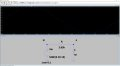The output is identical to the input, as it should be.

For 15kHz, the output magnitude is reduced by 70.71% which is correct, but this is not a filter, in my opinion, it should be zero from this point and forward: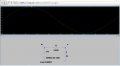For 20kHz, the output is still quite large: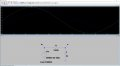And finally for 100kHz now the output approaches zero: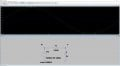So why is everyone on the Internet using this way to calculate R? How is this a good filter?

I tried mathematically to set the transfer function H(s) = 0 instead of 1/sqrt(2) but you can't solve for f or R because the result is infinity.

What if I set H(s) = something very small = 0.0000001? Will this work?
And even if this works, what exactly do we mean by very small? How can we define this small number?

Thanks!

#### Attachments

• 201.8 KB Views: 0
• 241.5 KB Views: 0
• 549 bytes Views: 0

#### crutschow

Joined Mar 14, 2008
31,126
So why is everyone on the Internet using this way to calculate R? How is this a good filter?
The normal definition for the filter frequency response (used long before the Internet) is the -3dB (half power) point or 0.707 amplitude of the passband frequency response, often called the "corner frequency".

No real filter has an instant cutoff (ideal brick wall filter) or zero response outside the passband as that's impossible.
The "goodness" of a filter is determined by how fast the response rolls off after the corner frequency, which is determined by how many poles the filter response equation has.
The response rolls off 8dB/octave or 20dB/decade of frequency beyond the corner frequency for each pole in the transfer response.

Below is the AC response simulation of your simple 15kHz RC filter.
The response is down -3dB at 15kHz as you noted, and rolls off 20dB/decade above that, as expected for a 1-pole filter.

Active filters with more capacitors and resistors, or passive filters with more inductors and capacitors to give more poles in the response, will give a faster rolloff.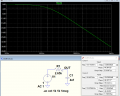Last edited:
•babaliaris

#### babaliaris

Joined Nov 19, 2019
158
Your answer is amazing! So the reason that setting Hs = 0 is impossible is that the answers that we get diverge right?

So making the circuit more complicated with more passive components I can achieve more poles in the denominator of the transfer function making it decay more quickly. I totally get that.

Now I need to understand what connections to add in the circuit (parallel or series) and which components to use in order to introduce more poles in the transfer function.

Do you know any sources that can help me understand more about designing filters?

There is a class by the way at my University but it's next semester, I can't waitLast edited:

#### WBahn

Joined Mar 31, 2012
27,892
This is done using the .ac command to perform a small-signal frequency analysis. By default, the plot is dB on the y-axis, relative to 1 V, and logarithmic on the x-axis.

His command basically tells LTSpice to perform an AC analysis, using 1000 points per octave (factor of two in frequency) starting with a frequency of 1 kHz and ending with a frequency of 1 MHz.

One thing to keep in mind when doing an AC simulation is that the circuit being simulated is the small-signal linearized version of the circuit and NOT the actual circuit. So you want to use a signal source that is 1 V to make the x-axis meaningful, but that does NOT mean that the actual circuit would respond to a 1 V signal this way -- that may be way too big a signal, for instance.

•babaliaris

#### SamR

Joined Mar 19, 2019
4,492

#### crutschow

Joined Mar 14, 2008
31,126
Do you know any sources that can help me understand more about designing filters?
A Google search turned up many.

I forgot to mention that another important characteristic of the filter is its damping factor, as that determines how fast it goes from the passband to the rolloff at the corner frequency.
For example, multipole passive filters made only with RC components will have a high damping factor with a slow transition, while LC filters with a lower damping factor will have a faster transition (sharp corner).

•babaliaris

#### Papabravo

Joined Feb 24, 2006
19,591
New textbook costs are crazy expensive so looking for previous editions of classic works is often a cost-effective way to go. I recommend:

Van Valkenburg, M. E., Analog Filter Design, Oxford University Press, 1982, ISBN 13 978-0-19-510734-0​

•babaliaris